# Electric Circuits

NOTE

This manual describes the laboratory experiment used during the 1996 - 1997 academic year. Significant changes have been made since then, and the manual used during the current academic year is in NOT available yet on the WEB. Hardcopies can be purchased at the bookstore.

# Electric Circuits

## Purpose

To learn about the concepts of capacitance, resistance, and inductance; and about the phenomenon of (electrical) resonance.

## Prelab Homework

This prelab homework must be done at home and handed to the lab TA before you start the lab. Read the instructions for this lab and consult the course textbook for relevant sections.

1. What is the voltage between the plates of a capacitor C when one puts a charge +Q on one plate and -Q on the other plate?

2. What is Ohm's law (i.e.. what is the relation between the voltage across a resistor and the current flowing through it)?

3. Consider a capacitor connected in parallel with a resistor. Suppose that it starts with a charge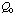at time t=0. Using the fact that the current I=dQ/dt, and using Ohm's law, derive the expression for the charge on the plates as a function of time.

4. What is the expression for the voltage between the plates as a function of time? (Also, see the appendix on Exponential Decay.)

## Introduction

This will be accomplished by first studying RC circuits and then resonant RLC circuits. To do this, you will first quickly review the use of an oscilloscope, the most versatile electronic measuring instrument. Then you will use this tool to investigate the characteristics of capacitors and resonant circuits.

### Review of the Oscilloscope

An oscilloscope is an instrument principally used to display signals as a function of time. With an oscilloscope it is possible to see and measure the details of wave shape, as well a,s qualities like frequency, period and amplitude. While these signals are primarily voltages, all manner of signals can be converted into voltages for observation.

The heart of an oscilloscope is a cathode ray tube (CRT), similar to that in a TV set, in which an electron beam excites a spot on a phosphor screen. The resulting visible spot of light is usually made to draw a graph where the y-axis is the measured signal and the x-axis is time. (But sometimes the x-axis is another signal so that the time behavior of the two can be compared.) An "electrostatic deflection tube" is used in which the electron beam is steered by two sets of plates that apply electric fields to deflect the electron beam in both the horizontal and vertical directions.

The voltage applied between the vertical or Y input and ground is amplified and applied to the vertical plates in the CRT to deflect the electron beam in the vertical direction. The deflection of the beam, and the corresponding deflection of the light spot, are proportional to the applied voltage. It is usually calibrated so that input voltage differences can be read directly from the vertical divisions on the screen according to the scale (amplification or gain) selected by the front panel control (Volts/Cm). This scale should be selected so that the height of the screen represents an amplitude a bit larger than the that of the input signal.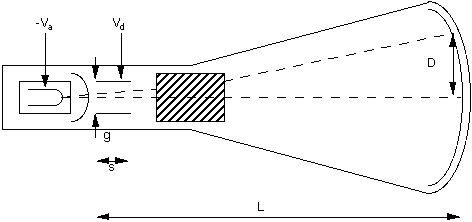Figure 10.1. Main elements of the Cathode Ray Tube(CRT)

In a similar manner the spot is deflected in the horizontal direction by a voltage applied to the horizontal plates. Usually this voltage is a "ramp" (that is a signal which increases linearly with time) generated internally by the oscilloscope's "time base" or "sweep generator." This sweeps the spot at a uniform, measured rate from the left side to the right side of the screen and then rapidly returns it to the left side to start over. In this way the horizontal position on the screen is proportional to time. The sweep rate is also usually calibrated so that time intervals can be read directly from the horizontal divisions on the screen according to the sweep rate selected by the (horizontal) front panel control (Time/Cm). This rate should be selected so that the width of the screen represents a time interval a bit larger than the duration of the features being investigated.

The result is that the oscilloscope plots out a graph of the input voltage as a function of time on the screen of the CRT. However, there are some practical details which must be understood in order to get satisfactory results. Familiarize yourself with your oscilloscope. Make sure it is plugged in. Find the controls discussed above. Disconnect any input cables and turn it on. Set it for free-running (or non-triggered) operation ("Auto" position or stability control clockwise); this will be explained below. You should see a horizontal line (the graph of a constant zero volts input). Make sure the vertical and horizontal positions are on the screen and adjust focus and intensity to give a satisfactory display. If necessary to get started, ask your instructor for assistance. Try adjusting the horizontal sweep control (Time/Cm) through its range to observe its effect.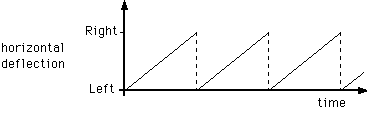Figure 10.2. Horizontal Sweep Ramp

If the voltage changes were slow enough then on a very slow sweep speed you could directly see the progress of the spot around the screen. Electronic signals are often too fast to see this way. Besides, it is hard to see signals slower than the persistence of the phosphor because, as the beam moves on, the illuminated trace fades from view; this prevents you from seeing the whole wave form at once.

So, for reasons of practicality and convenience, the spot will usually be moving too rapidly for your eye to follow. What you actually see will be the superposition of multiple successive tracks across the screen. Only if the tracks exactly coincide will the image you see be a single sharp line. Otherwise, it will be rather unintelligible.

This will not be possible unless the input signal is periodic. Even then it will not occur if the period of the signal does not happen to be the exactly the same as that of the sweep (called synchronization). This situation is illustrated in the Figure 10.3.

One way to accomplish synchronization is to adjust either the frequency of the input signal or of the sweep so that they match. This is often hard to do satisfactorily because things tend to drift; besides it may be experimentally inconvenient.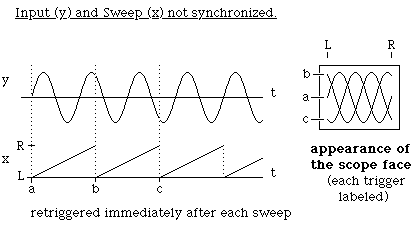Figure 10.3

Connect a function generator to an input of the oscilloscope (check that the input channel is turned on). Set it for sine wave output and about 1000Hz frequency with the amplitude control at mid-range. Find the appropriate setting for the time base and the vertical gain. Make sure the oscilloscope is in free-running mode and attempt synchronization both by adjusting the frequency of the signal generator and by using the variable (non-calibrated) sweep rate control. You should observe the situation described above.

A more satisfactory solution to the synchronization problem is provided by "triggered" operation of the oscilloscope. In this mode, rather than having the ramp start over as soon as the previous sweep is finished (called "free-running"), the time base waits to restart the ramp until the input crosses a certain voltage level ("triggers"). As long as this is the same point of the input wave form each time, the sweep across the screen will be synchronized with the repeating signal. This is illustrated in the Figure 10.4.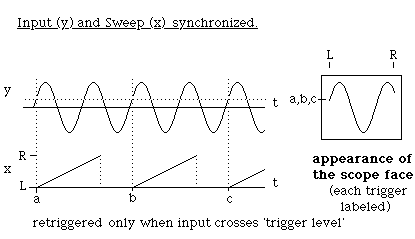Figure 10.4

To use triggered operation, stop the sweep from free-running (set to "Norm" or turn the stability control counter-clockwise to near mid-range). Set the trigger selector to internal and activate the trigger button for the channel from which you want to trigger. Adjust the trigger level to stabilize the display. Observe what happens to the display as you vary the trigger level and change the sign of the trigger selection. (The auto position of the trigger level causes triggering to occur at the mean level of the input wave form.) Change the amplitude of the generator and note how the display changes.Measure the amplitude and frequency of the signal. To do this accurately, the variable controls on the time base and the vertical gain must be in the "cal"(ibrated) position. Observe the square and triangle outputs of the signal generator also.

On Tel equipment scopes only: the stability control allows three modes of triggering:

1. Fully clockwise: triggering occurs continuously (free-running).

2. Fully counterclockwise: no triggering occurs.

3. Midrange: sweep will trigger on the level you set with the trigger level control. Normally this control should adjusted just to the point at which triggering begins.

When using both channels, keep the alt.-chop switch in the alt. position.

Caution:

1. Don't let the trace exist as a dot on the screen; it can damage the phosphor.

2. Don't look at big (>50V) voltages (i.e., the AC line).

3. All signals, both input and output, are with respect to a common ground. This is a real earth ground through the chassis and the third prong of the AC plug. The shields of all the BNC connectors are connected together and to this ground and the input signals are applied between it and the center terminal of their BNC. connectors. Thus, when using the scope you must not do anything inconsistent with this situation (like trying to apply a voltage between the shields of two different inputs).

## Part I: RC Circuits

A capacitor stores electric charge. Typically, a capacitor is made of two parallel plates. Each plate will have a charge of the same magnitude Q but of opposite sign between which there will be an electric field. The work (per unit charge) this field would do on a test charge moved between the plates is the potential difference or voltage V across the plates of the capacitor.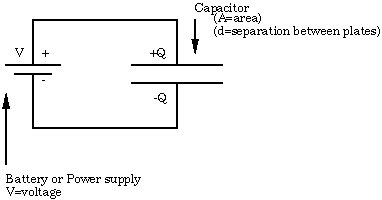Figure 10.5

The charge and voltage are linearly related by a constant C which measures the capacitance. Consider the situation in which a capacitor is charged by a battery through a resistance as shown below (switch in the charge position). The charge stored on the capacitor (and therefore the voltage across it) increases with time because of the current, which is a flow of charge through the resistance. This current, by Ohm's law, is proportional to the voltage across the resistor,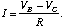(10.1)

This current will decrease as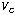rises toward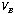so the rate at which the capacitor is charging up will fall. Eventually, when there is no further flow of charge the capacitor will be fully charged to its final value,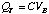(10.2)

The charge on the capacitor and the voltage across the capacitor do not grow linearly with time. Rather, they follow an exponential law. If the capacitor is initially (at t = 0) discharged this takes the form (see Appendix D on Exponential Decay),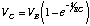(10.3)

After a time t = RC, the capacitor is charged to within,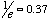of the final value. The value RC is known as the time constant of the circuit. If the resistance R is in ohms and the capacitance C is in farads then the time constant RC is in seconds.Figure 10.6. RC Circuit

Similarly, if a fully charged capacitor is discharged through a resistance (e.g. by moving the switch to the discharge position) the voltage across the capacitor (and the charge on it) will fall to zero exponentially with time, as shown below,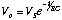(10.4)Figure 10.7. Charging a Capacitor through a Resistor

Again, after a time t = RC the capacitor will discharge to 0.63 of its initial value.

In the first part of this experiment you will investigate the charging and discharging of a capacitor for different values of the time constant. By observing the voltage on the capacitor with an oscilloscope you can measure it as a function of time.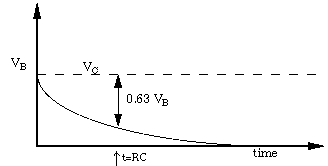Figure 10.8. Discharging a Capacitor

Although for purposes of illustration it is convenient to discuss charging and discharging the capacitor with a switch, it is experimentally more practical to apply a square wave which duplicates the switch on and off action with a signal generator as shown below. This is a way of regularly switching the applied voltage between two values so that the capacitor can charge and discharge between them. The charging and discharging traces will be accurately reproducible from cycle to cycle. Then if the oscilloscope is triggered off the periodic input these successive traces will overlay each other on the screen. And the "switching" can occur at a fast enough rate that the result will be a bright, stable display from which you can make measurements. If the period of the square wave is over several time constants the capacitor will approach very close to its final value before the square wave switches.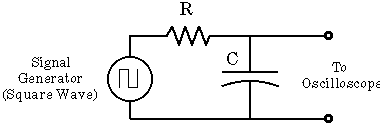Figure 10.9. RC Test Circuit

Hook up the function generator, set to produce square waves, as above using (variable) resistor and capacitor decade boxes. Find a convenient amplitude and frequency and then look at and trigger on the output of the signal generator. Connect the other channel to observe the capacitor voltage at the same time. You should obtain a display similar to that shown in Figure 10.10.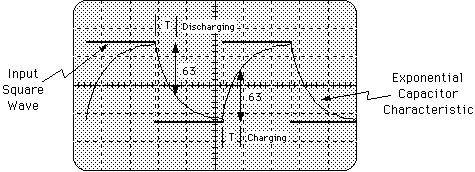Figure 10.10

Data sheets are available on which to record your data. (See sample data sheets at the end of this lab). Complete them for the experiments below before you leave and hand them in with your lab report.

### Procedure

1. Set the decade boxes to the values R=3,000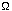; C=0.01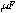and the frequency of the square wave (=3000Hz). Adjust the frequency of the signal generator for an appropriate display (see figure 10.10) and trace it on a data sheet. Determine both the charging and discharging time constant by measuring the time taken for the voltage to change from its initial value to within 1/e of its final value.

2. Compare the charging time constant and the discharging time constant with the theoretical value of R x C.

3. Change the value of R or C by 30% and repeat the above measurements.

4. Get an unknown capacitance from the TA and substitute it for the capacitance decade box. Adjust the resistor and the signal generator frequency for a convenient display and measure the time constant as above. Use the result to obtain an experimental value for the capacitance.

5. Get an unknown resistor from the TA and determine its value in a manner similar to the above.

### Questions

1. What systematic or random errors can you think of which might account for differences between the measured and calculated values of the time constants?

2. How accurately do you think you can measure the time constant from your tracing?

3. What is the result of the fact that with a finite period there is not enough time for the capacitor to charge all the way to its final value?

## Part II: The Forced Damped Oscillator

In this section you will study the electrical version of the mechanical system that you have studied in your mechanics course. There is an exact isomorphism between the two cases as Figure 10.11 indicates.

On the right we have a mass m on a spring of constant k and with damping b. This is being driven by an oscillating force of constant amplitude at an angular frequency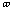(which can be varied). This system has a resonant frequency,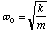(10.5)

for which the response is a maximum when the driving frequencyis equal to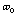. In the vicinity ofthe response curve looks like Figure 10.12,

Where the width of the curve (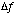) from the point where A =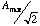=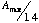is given by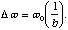There is a demonstration of a mechanical system which your teaching assistant will show you.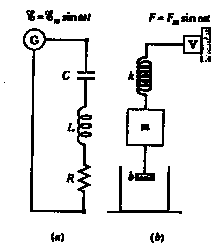Figure 10.11. (a) is the electrical system, (b) is the mechanical system.

### Introduction

In this experiment you will investigate the general phenomenon of resonance in the form of the particular example of an RLC (resistor, inductor and capacitor) circuit. You will be able to determine steady state behavior as well as the Q (quality) factor. In the process you will gain some experience with electronic circuits and components. You will set up the circuit shown in figure 10.13. Be sure that the ground to the signal generator is the same as the voltmeter.

The basic equation that describes the phenomenon of resonance is that of a driven, damped, harmonic oscillator. In the case of the above RLC circuit, this takes the form,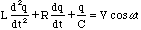(10.6)

where q is the charge on the capacitor and V is the peak amplitude of the signal generator and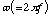is the angular frequency.

It is important to understand that the phenomena are characteristic of the equation and not peculiar to its electrical realization. For instance, if it were a mechanical oscillator the phenomena of resonance would be the same.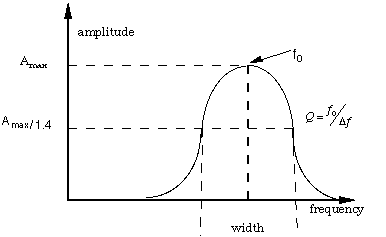Figure 10.12

### Theory on Steady State RLC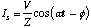(10.7)

where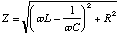(10.8)

and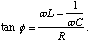(10.9)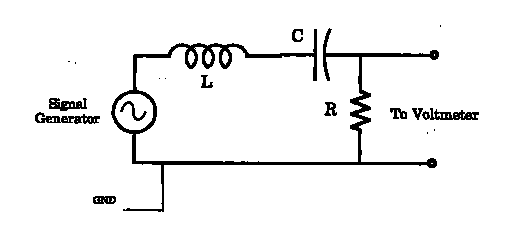Figure 10.13. A Driven RLC Circuit

The general solution of this equation (written in terms of I = dq/dt, which is more practical to measure) has two parts,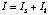, a steady state and a transient term.

You are going to measure the RMS voltage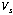across the resistor. So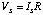. The steady state Is is the term caused by the driving voltage; it is all that is left after initial transients have died away. The physical significance of these quantities can be made more apparent by expressing them in terms of the more universal quantities resonant angular frequency,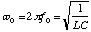(10.10)

and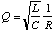(10.11)

the quality factor Q is roughly the number of oscillations it takes for the transient to die down. Thus in terms ofand Q we have,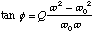(10.12)

and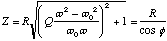(10.13)

it is apparent that, in the steady state, at the resonant frequency (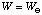) the phase angle will be zero, the circuit will act just like a resistance R and the current will be maximum. Away from the resonant frequency the amplitude of the current will decrease. The "full width at half maximum (half power = .707 of maximum current)" of the resonance curve will be about,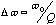(10.14)

that is it will be narrower, in terms of a fraction of the resonant frequency, directly as Q increases.

### Procedure

Assemble the experimental circuit above using the values: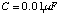,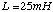,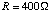1. Calculate the resonant frequency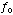. Set the oscillator frequency f near the value calculated. The oscillator voltage should be set at around 3 Volts.

2. Measure the output with the voltmeter.

3. Now vary the frequency f to find the maximum voltage,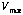. This frequency will probably be a little different from your calculation. You do not need the oscilloscope for this part of the experiment. The curve nearshould look like that in figure 10.12 Take measurements of V in the vicinity of, two measurements below and above will be sufficient.

4. Now change R to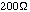and repeat the measurements.

5. Now change R to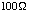and repeat the measurements. At this point you have done three sets of measurements.

6. Now change the values to C=0.01F, L=100H,. Now find the resonant curve. Is the resonant frequency one half of what it was in the above experiments?

7. Repeat for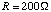.

8. Now change R toand repeat. (You should have six data sets.)

### Data Analysis

1. Is the resonant frequency independent of R as expected?

2. Measure theof each of the curves.

3. Plot 1/Q vs. R for the C=0.01f and L=25mH data. Is this a straight line as expected?

4. Do the same as 3) for the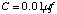, L=100mH data.

Capacitance

Date:_________________________

Name:____________________________________

Data Sheet for known: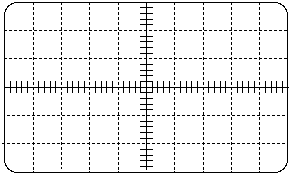______________________seconds(measured T charging)

______________________seconds(measured T discharging)

______________________ohms

R

______________________ohms

C

______________________ohms

RC

Capacitance

Date:_________________________

Name:____________________________________

Data Sheet for known: 30% values______________________seconds(measured T charging)

______________________seconds(measured T discharging)

______________________ohms

R

______________________ohms

C

______________________ohms

RC

Capacitance

Date:______________________________

Name:__________________________________

Data Sheet for unknown C______________________seconds(measured T charging)

______________________seconds(measured T discharging)

______________________ohms

R

______________________ohms

C

______________________ohms

RC

Capacitance

Date:_________________________

Name:____________________________________

Data Sheet for unknown R______________________seconds(measured T charging)

______________________seconds(measured T discharging)

______________________ohms

R

______________________ohms

C

______________________ohms

RC

Date:______________________________

Name:_____________________________

Initial Values: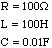Frequency Voltage

Date:______________________________

Name:_____________________________

Initial Values:Frequency Voltage

Date:______________________________

Name:_____________________________

Initial Values:Frequency Voltage

Date:______________________________

Name:_____________________________

Initial Values:Frequency Voltage

Date:______________________________

Name:_____________________________

Initial Values:Frequency Voltage

Date:______________________________

Name:_____________________________

Initial Values:Frequency Voltage

Data

Sine Wave

(Suggested values for RLC in Resonance)

 Capacitance (nF) Resistance (K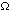) Inductance (mH) Frequency (KHz) Max .043nF Min .028nF 3K30mH 105KHz Max .326nF Min .046nF 13K30mH 250KHZ Max .058nF Min. .030nF 3K30mH 90KHz Max .264nF Min .036nF 3K25mH 70KHz Max .392nF Min .048nF 3K50mH 50KHz Max .32nF Min .04nF 3K100mH 25KHx Max .321nF Min .041nF 3K100mH 15KHz Max .323nF Min .041nF 3K100mH 25KHz Max .327nF Min .043nF 3K100mH 80KHz

Data

Square Wave

 Capacitance (nF) Resistance (K) Inductance (mH) Frequency (KHz) unchanged 13K& up unchanged 1.5KHz unchanged 52K& up unchanged 2.3KHz unchanged 20K& up unchanged 2.4KHZ unchanged 13K& up unchanged 1.1KHZ unchanged 13K& up unchanged 8KHz unchanged 13K& up unchanged 2KHz unchanged 13K& up unchanged .3KHz unchanged 13K& up unchanged .8KHz unchanged 13K& up unchanged .9KHz